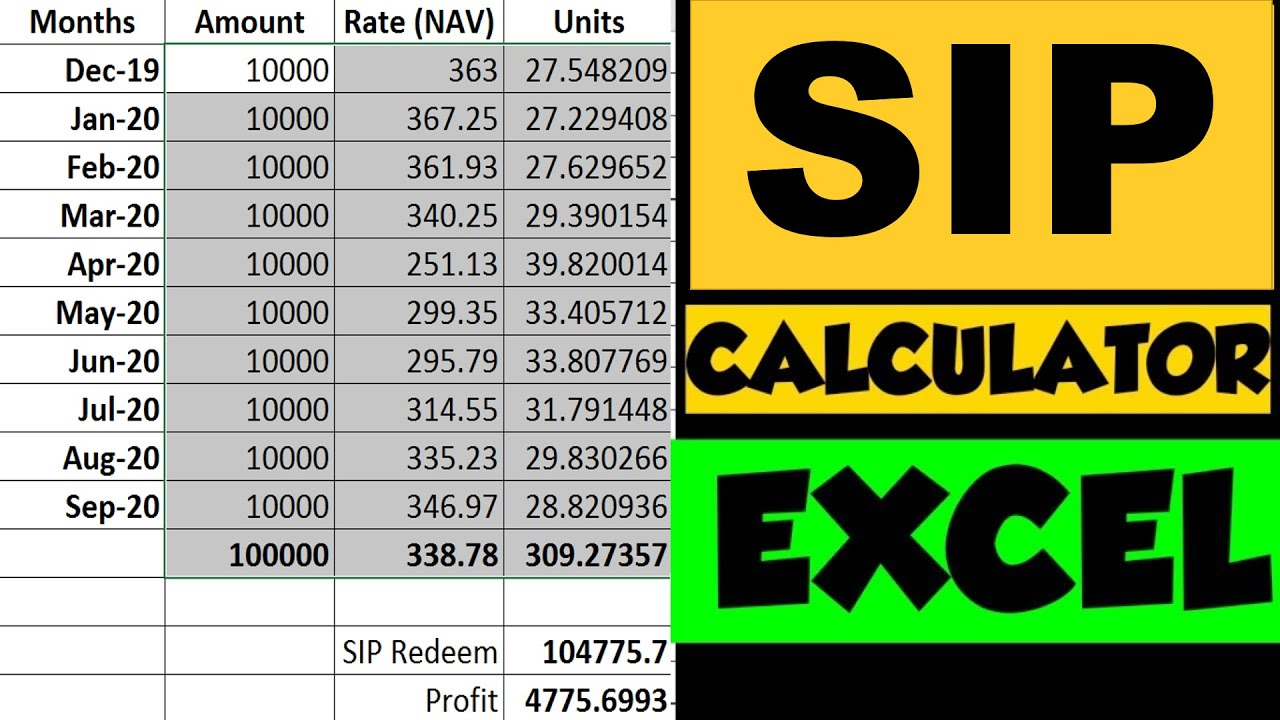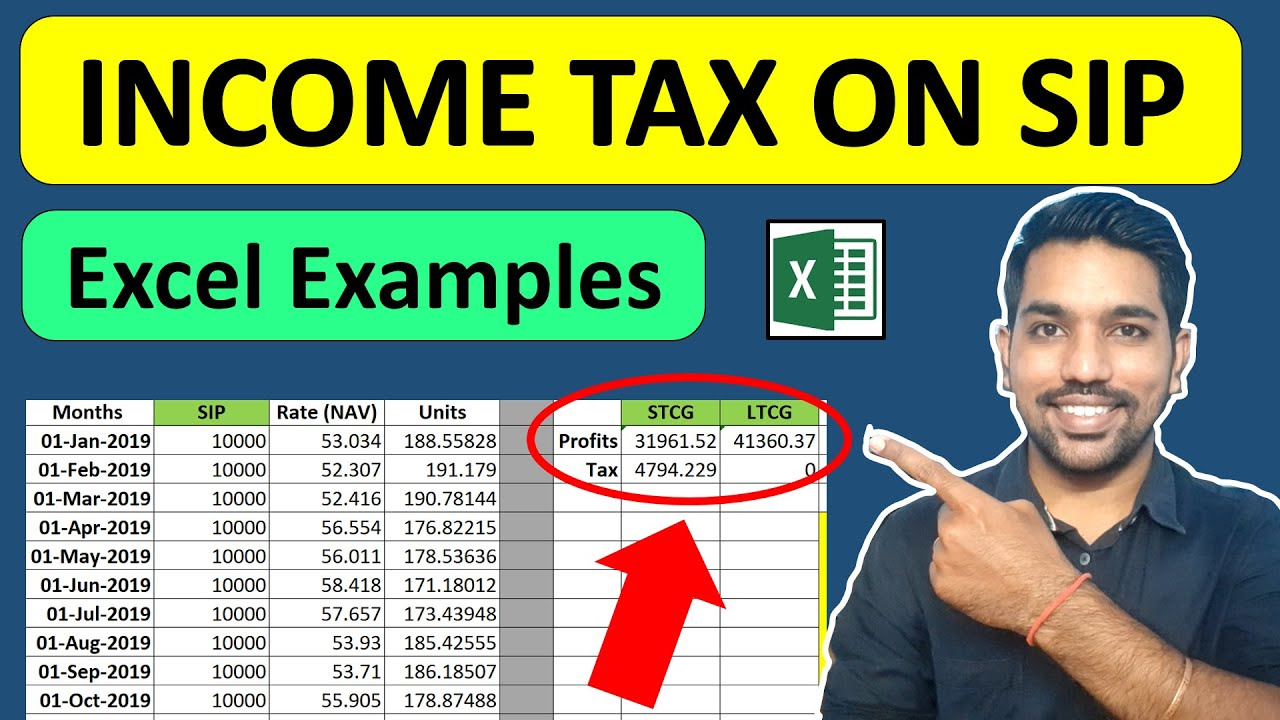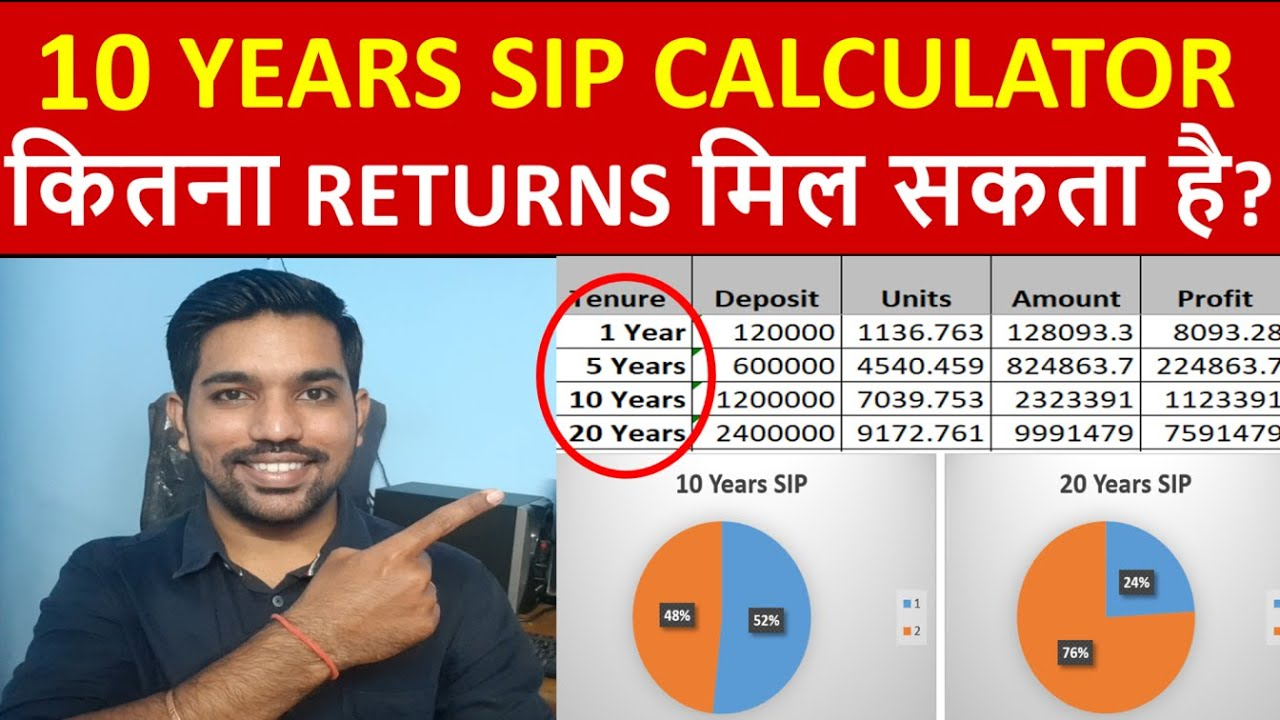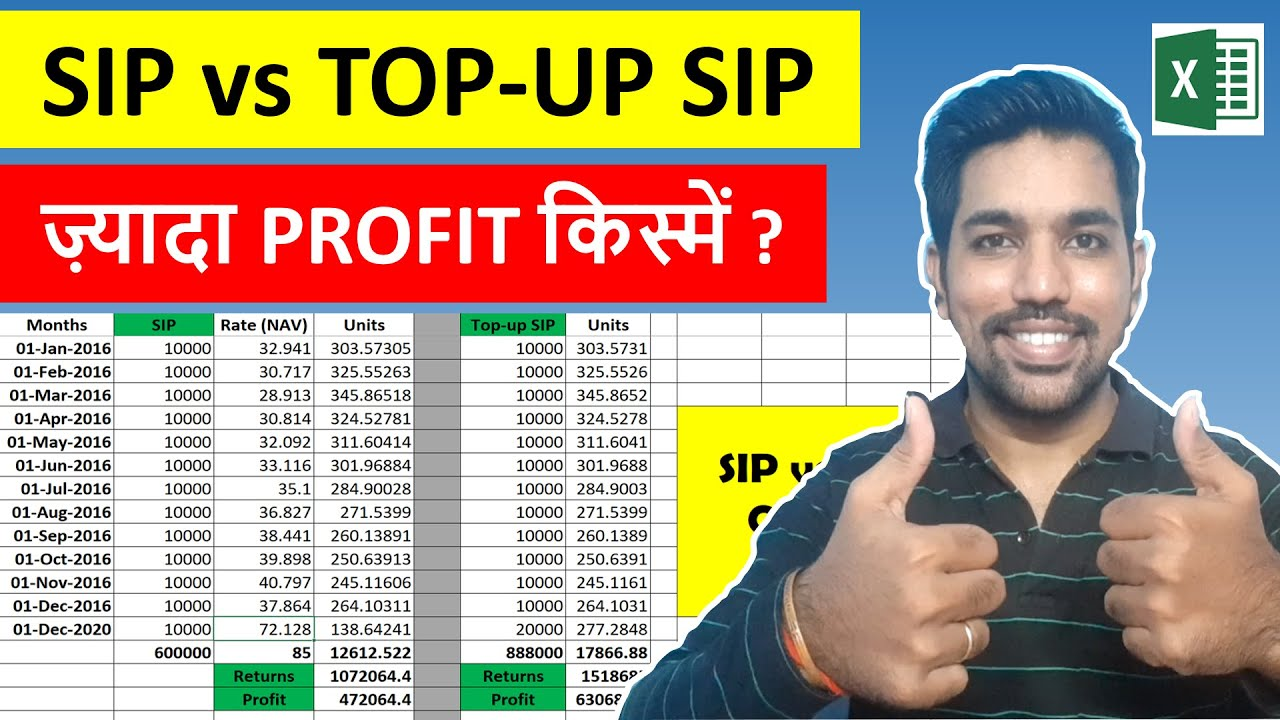# SIP Calculation in Excel | How to Calculate SIP Returns [Examples]We can easily perform SIP calculation in excel in case we want to understand more about every month returns from our mutual funds SIP investments, track the investments on monthly basis and easily find rate of return on our investments.

The process to calculate SIP returns in excel include tracking the NAV (Net Asset Value) of a mutual fund in which SIP (Systematic Investment Plan) is being done, calculate number of units by dividing the amount invested by the NAV value on the day of investment, adding all the units we have accumulated in the past and then multiplying the total accumulated units by the current value of NAV. This will show us the current value of investments in excel. Also, we can use the SIP excel calculator to calculate future returns of mutual fund SIP investments.

We will see all this with the help of examples.

You can also use our online SIP calculator to quickly calculate your SIP returns.

## What is Mutual Funds SIP?

SIP full form is Systematic Investment Plan. SIP is a way to invest in mutual funds or any investment option every month regularly. In this article we will consider SIP as investments in mutual funds for simplicity. You can also do SIP in Stocks or Shares of companies.

So, why SIP is important and why you should start SIP if you have not started yet? Before moving to the actual reasons of investing via SIP, let’s see some stats.

In 2016-17, total monthly SIP collections were 43,921 crore which grew to Rs. 1,00,800 by FY 2021-22. That’s more than 100% in just 5 years.

Also, currently there are 5.05 crore SIP accounts in India (January 2022) that are actively investing in various mutual funds via SIP.

Now what makes people of India show so much interest in mutual funds SIP? It’s because of SIP’s unique features such as Rupee cost Averaging and the reduction in amount of risks compared to lump sum investing in mutual funds or stocks.

Below are some benefits of SIP:

1. Rupee Cost Averaging: This is the term derived from dollar cost averaging but let’s understand this in terms of rupee! The fact that SIP invests in mutual funds regularly, helps you to buy less mutual funds units when market goes up (price goes up) and more mutual funds units when market goes down (price goes down). We will see this in examples below.
2. Lowering risk: As compared to lump sum investing where you invest entire amount in one go after seeing a small drop in market, your SIPs help you to balance your investment value by seeing the ups and downs of market while helping you invest regularly. This lowers the risk in SIP.
3. Mental Strength: In SIP you do not pay huge amount up front like lump sum investing. You always pay a fraction of amount which you can afford to invest and reap benefits over long term.
4. Achieving goals: You must have heard about – “Slow and steady wins the race“. It’s not about the sprints that you take but it’s about the marathon that you win slowly and with patience. SIP helps in achieving your long term goals by keeping aside some amount of money every month.

Below video helps you in understanding SIP returns calculation:Watch more Excel Videos Here

## What is NAV value in SIP?

Now since you have understood what is SIP and what are the benefits of SIP, you should know what is NAV of a mutual fund and it’s importance.

NAV full form is Net Asset Value, which is the price of single unit of a mutual fund. Let’s say when you buy an egg for Rs. 5, that’s the price of 1 quantity of egg. If you buy 6 eggs (half dozen), you have to pay – 6 quantity * Rs. 5 per quantity price = Rs. 30.

Similarly, there is NAV value of a mutual fund. It varies for different mutual funds since there are several mutual funds in market. Just like we don’t get egg and slice breads for same price, mutual funds also have different NAV values.

It is a myth that you should choose mutual funds based on their NAV values. You don’t buy all eggs if slice bread price is more than eggs. You need both so you buy both. Similarly you should not select mutual funds based on their NAV values, but based on your goals you want to achieve.

You can also see the top mutual funds you can choose from, based on your risk appetite and goals.

## How do you calculate SIP in Excel?

Now since you have got the idea about what is SIP and and what is NAV in mutual funds, let us now understand how to calculate SIP returns using excel. As I said, SIP calculation in excel is very easy and you can track your investments by yourself.

Step 1: We will take an example of Mutual fund for which I have tracked the NAV values of 1 year. Let’s consider SIP amount of Rs. 5000 that we will invest every month. Below is the how the figures will look like in Excel:

Step 2: Next, we calculate the number of units for each month’s investment by using the units calculation formula – Units = Amount / NAV. This calculation will be applied for every month’s SIP installments as shown below:

Step 3: Now if we want to sell our mutual funds units (redeem funds) we can sell based on the latest NAV value of Mutual fund. Below is the excel image for total units calculation (all units added from each month) and then selling all units based on latest NAV value by using formula – Total Amount = total units * latest NAV

As you can see for above example, we get a profit of Rs. 18,024 based on above SIP investments.

## When your SIP gets Matured?

It is important to mention here that you can sell your mutual fund units when doing SIP, anytime you want. There is no SIP maturity defined. There is no compulsion to keep your SIP investments for 1 year or 5 years or any specific period. You are free to buy and sell anytime you want.

But during selling, there are some conditions based on mutual fund selected that they need you to stay invested for a specific period at least. Which we call as Exit criteria.

One exit criteria can be defined as – “0.1% charged for units redeemed in less than a year”. This means that if you buy units and sell them within 1 year, you will be charged 0.1% of the profits you made from those units.

Now in SIP, every month’s units will have their own 1 year period to be completed based on exit criteria. But still with this exit criteria, you can remove the funds within one year by giving up 0.1% of your profits (if you are ok with that), which will be a negligible amount from your profits.

But if you can wait and hold the units for more than a year, no charges will be applied to your redemption request or when you sell the mutual funds units.

ALSO READ: SWP Calculator with Inflation | Systematic Withdrawal Plan

More Recommended Books Here

## What is the formula for calculating SIP in Excel?

As seen in above example of SIP calculation n excel, there are few formulas you should know while you calculate your SIP returns in Excel.

1. First mention the months, NAV values of mutual funds and SIP amounts in first 3 columns in excel
2. Using the formula of Number of Units = Amount / NAV, we get units for all months mentioned
3. We add all units of every month to reach sum of units in the month we want to sell mutual funds units
4. Based on the latest NAV value we use the formula – Total Amount = Total Units * Latest NAV, and get the total maturity amount of our SIP investment
5. We than find the difference between maturity amount and total amount invested to find the profits we made

This is the way we find how much profits we made in SIP. But these profits are taxable when your LTCG or long term capital gains is above Rs. 1 Lac in a FY. You can check How Income Tax is calculated on SIP here.## Calculating future value of SIP in Excel

The above example we saw about an existing mutual fund with past NAV values and calculating current value by reaching the total maturity amount and profits.

But can we calculate the future value of our SIP investments? Based on expected returns in the range of 10% to 15% a year that we get in mutual funds, can we calculate the total maturity amount after 10 years or 20 years?

The answer is yes. We can use another format of excel by mentioning the expected returns in the mutual fund and calculate returns based on this expected returns.

Since we don’t have the NAV values of future, we use the yearly expected returns and calculate the expected NAV values first, and then the rest of the steps are same as mentioned above. Below are the steps to calculate the future value of SIP investments:

1. Mention the number of months and yearly expected returns for every month in excel
2. Provide the initial value of NAV (for first month), and based on the yearly expected return, calculate next month NAV value. Formula to calculate current month NAV – NAV = (yearly Expected return / 12 * Previous month’s NAV / 100) + Previous month NAV. This will give us expected NAV value based on expected return we mentioned
3. Repeat step 2 for remaining months of NAV values
4. Once we have all NAV values, we can calculate number of units for all months. Using the formula of Number of Units = Amount / NAV, we get units for all months
5. We add all units of every month to reach sum of units in the month we want to sell mutual funds units
6. Based on the latest NAV value we use the formula – Total Amount = Total Units * Latest NAV, and get the total maturity amount of our SIP investment
7. We than find the difference between maturity amount and total amount invested to find the profits we made

Below is the video for which we calculated next 10 years SIP returns using above steps:You can also calculate 20 years SIP returns in mutual fund in similar way!

## HOW IS SIP TOP UP CALCULATED?

SIP top up also known as Step up SIP is a way to increase your SIP investment amount regularly (usually after a year), when you get salary increments, promotions or bonuses. This is the effective way to increase your investment amount so that you reach your goals before time.

I have explained the difference between SIP and Step up SIP here, where you will see the step up SIP has more advantages apart from the advantages of SIP we already saw.

With discipline, step up SIP helps us achieve our goals before time, if we can afford to increase our SIP amount regularly and many mutual fund companies provide us the option of increasing our investment amount every year or quarter with the step up SIP option.

Below is another video on STEP up SIP vs normal SIP comparison of returns and benefits:Enter Correct Email ID to receive Excel
If you opt in above, we use this information to send related contentSo that’s all in this way of SIP calculation in Excel. Hope you have learnt many things about SIP and its’ benefits in this article, along with informative videos I have provided. You can also Download my free Android App “FinCalC” to access various calculators and help yourself maintain financial stability with required personal finance knowledge.

Found this Helpful? DONATE any amount to see more useful Content. Scan below QR code using any UPI App!

UPI ID: abhilashgupta8149-1@okhdfcbank

Verify that you are “Paying Abhilash Gupta” before making the transaction so that it reaches me. It makes my Day 🙂

Thank you for Donating. Stay Tuned!

## Income Tax Calculator App – FinCalC

For Income Tax Calculation on your mobile device, you can Download my Android App “FinCalC” which I have developed for you to make your income tax calculation easy.

### What you can do with this mobile App?

• Calculate Income Tax for new FY 2023-24 and previous FY 2022-23
• Enter estimated Investments to check income tax with Old and New Tax Regime
• Save income tax details and track regularly
• Know how much to invest more to save income tax
• More calculators including PPF, SIP returns, Savings account interest and lot more

How to Become Crorepati with SIP investments

How Compounding is SIP works

SIP & Lump sum returns Calculator for Next 30 Years

Use Popular Calculators:

I’d love to hear from you if you have any queries about Personal Finance and Money Management.

JOIN Telegram Group and stay updated with latest Personal Finance News and Topics.

Download our Free Android App – FinCalC to Calculate Income Tax and Interest on various small Saving Schemes in India including PPF, NSC, SIP and lot more.